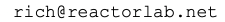# Web Labs now have the capability of desktop Reactor Lab

With the addition today of Web Lab 13, batch reactor with n-th order reaction, Web Labs now have examples of all the types of labs and plots in the desktop Reactor Lab.

In Dynamic lab types, the simulation runs continuously in time, with the student being able to change inputs during the simulation. In non-dynamic labs, individual experiments are run, which produce one or more plots for that experiment. In quizzes, such as the current Lab 7 Quiz, students can run and analyze experiments to determine unknown input values.

Plot types include profile, single, strip, and color canvas. A profile plot shows how an output has changed with time or space. Single type plots show results of non-dynamic experiments as x,y points as inputs are changed between experiments, with the student being able to select which inputs and outputs are shown on both the x and y axes.

A strip plot is like a lab strip chart (do they have those anymore?) which continuously scrolls in time as outputs are plotted. A color canvas plot shows how a color-coded output varies in two dimensions, e.g., time and space.

Graphics can also be dynamically drawn with SVG graphics, such as in Web Lab 00, a simulation of a pendulum. Several labs change the size and color of HTML div elements to show fluid levels and concentrations changing.

Do you have any suggestions for new labs? Please let us know by sending email to